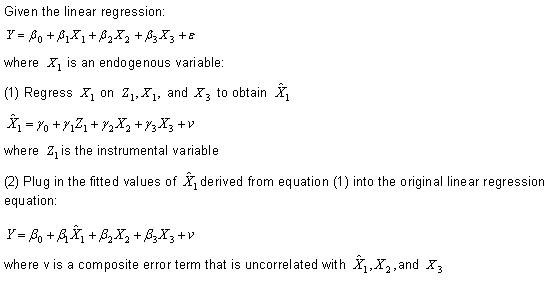## 2SLS regression analysis (Two-Stage least squares): Instrumental Variables (IV) #Endogeneity #SPSS #R #STATA#### Two-Stage Least Squares (2SLS) Regression Analysis

Two-Stage least squares (2SLS) regression analysis is a statistical technique that is used in the analysis of structural equations. This technique is the extension of the OLS method. It is used when the dependent variable’s error terms are correlated with the independent variables. Additionally, it is useful when there are feedback loops in the model. In structural equations modeling, we use the maximum likelihood method to estimate the path coefficient. This technique is an alternative in SEM modeling to estimate the path coefficient. This technique can also be applied in quasi-experimental studies.

How much can be budgeted in order to accurately estimate how much wheat is needed to produce bread?

What is the price of wheat? Is it on an upward trend?

Determine the final price for its bread.

Assumptions:

1. Models (equations) should be correctly identified.
2. The error variance of all the variables should be equal.
3. Error terms should be normally distributed.
4. It is assumed that the outlier(s) is removed from the data.
5. Observations should be independents of each other.Key concepts and terms:

Problematic causal variable: The dependent or endogenous variable whose error term is correlated with the other dependent variable error term.  A problematic causal variable is replaced with the substitute variable in the first stage of the analysis.

Instruments: An instrument variable is used to create a new variable by replacing the problematic variable.

Stages: In ordinary least square method, there is a basic assumption that the value of the error terms is independent of predictor variables. When this assumption is broken, this technique helps us to solve this problem.  This analysis assumes that there is a secondary predictor that is correlated to the problematic predictor but not with the error term.  Given the existence of the instrument variable, the following two methods are used:

1. In the first stage, a new variable is created using the instrument variable.
2. In the second stage, the model-estimated values from stage one are then used in place of the actual values of the problematic predictors to compute an OLS model for the response of interest.

SPSS:

All statistical software does not perform this regression method.  In SPSS, to perform this analysis, the following steps are involved:

1. Click on the “SPSS” icon from the start menu.
2. Click on the “Open data” icon and select the data.
3. Click on the “analysis” menu and select the “regression” option.
4. Select two-stage least squares (2SLS) regression analysis from the regression option.  From the 2SLS regression window, select the dependent, independent and instrumental variable.  Click on the “ok” button. The result window will appear in front of us.  The result explanation of the analysis is same as the OLS, MLE or WLS method.

STATA:

R:

Sources:

http://www.statisticssolutions.com/two-stage-least-squares-2sls-regression-analysis/

Endogeneity: An inconvenient truth (for researchers), by John Antonakis